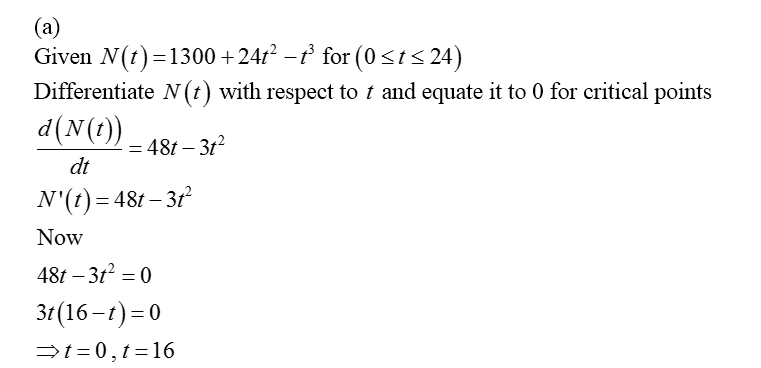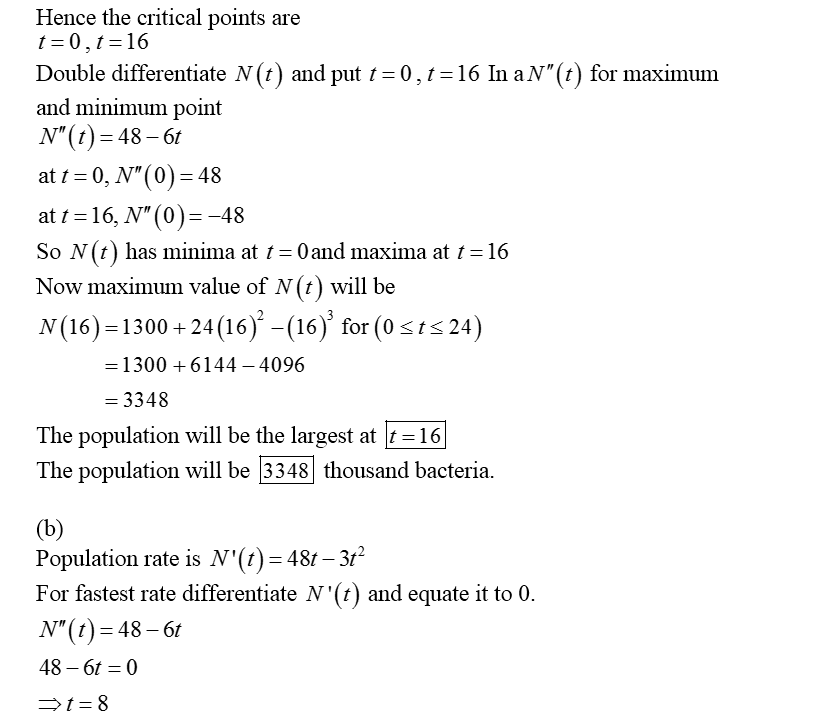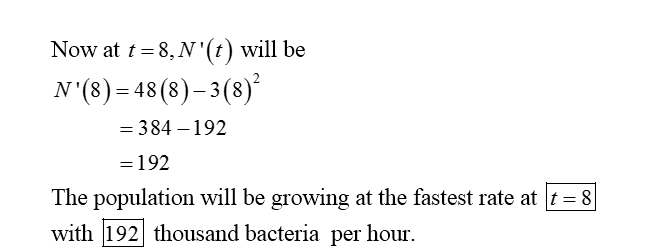# A chemical reagent is introduced into a bacterial population, and t hours later the number of bacteria (in thousands) is N(t) = 1300 + 24t2 − t3 (for 0 ≤ t ≤ 24).(a) When will the population be the largest?t = How large will it be? thousand bacteria(b) When will the population be growing at the fastest rate?t = How fast? thousand bacteria  per hourWhat word applies to such a point?a)minimum pointb)inflection point    c)rate pointd)maximum pointe) critical poin

Question
14 views

A chemical reagent is introduced into a bacterial population, and t hours later the number of bacteria (in thousands) is

N(t) = 1300 + 24t2 − t3
(for 0 ≤ t ≤ 24).
(a) When will the population be the largest?
t =
How large will it be?
thousand bacteria
(b) When will the population be growing at the fastest rate?
t =

How fast?
thousand bacteria  per hour

What word applies to such a point?
a)minimum point
b)inflection point
c)rate point
d)maximum point
e) critical poin
check_circle

Step 1Step 2Step 3...

### Want to see the full answer?

See Solution

#### Want to see this answer and more?

Solutions are written by subject experts who are available 24/7. Questions are typically answered within 1 hour.*

See Solution
*Response times may vary by subject and question.
Tagged in

### Calculus